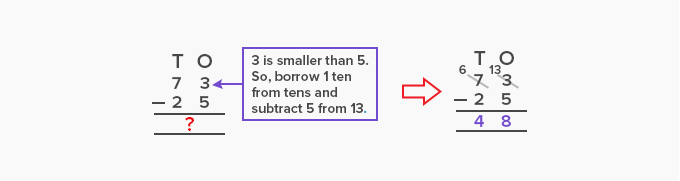# Minuend - Definition with Examples

The Complete K-5 Math Learning Program Built for Your Child

• 30 Million Kids

Loved by kids and parent worldwide

• 50,000 Schools

Trusted by teachers across schools

• Comprehensive Curriculum

Aligned to Common Core

What is Minuend?

The number in a subtraction sentence from which we subtract another number is called a minuend.

A subtraction sentence consists of 3 numbers:

Minuend, Subtrahend and Difference

Minuend is the first number in a subtraction sentence. We subtract subtrahend from the minuend to get the difference.In vertical method of subtraction or column method, the minuend is the number on the top.In the column method of subtraction, we regroup the numbers if the minuend is smaller than the subtrahend, that is, the number we want to subtract is higher than the number from which it is subtracted. In such a case, we borrow 1 from the next higher place to regroup the numbers. It makes the value of minuend higher than the subtrahend and makes it possible to subtract the subtrahend from the minuend.Here, 5 cannot be subtracted from 3. So, 1 ten is borrowed from the tens place. This changes the minuend from 3 to 13. Now, 5 can be easily subtracted from 13 to get 8.

 Fun Facts The word “minuend” originates from the Latin word “minuendus”, which means something that will decrease.   In a subtraction sentence, the value of the minuend is equal to the sum of the subtrahend and the difference. For example, if _____  – 7 = 28; then the minuend is 28 + 7 = 35.
Won Numerous Awards & Honors